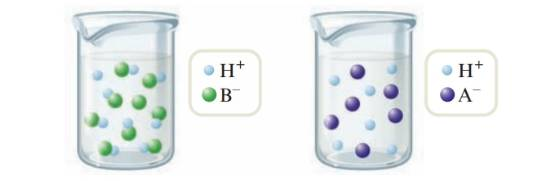Chapter 16, Problem 16ALQ### Introductory Chemistry: A Foundati...

9th Edition
Steven S. Zumdahl + 1 other
ISBN: 9781337399425

#### Solutions

Chapter
Section### Introductory Chemistry: A Foundati...

9th Edition
Steven S. Zumdahl + 1 other
ISBN: 9781337399425
Textbook Problem
1 views

# . The following figures are molecular-level representations of acid solutions. Label each as a strong acid or a weak acid.Interpretation Introduction

Interpretation:

Each representation of acid solutions should be labeled as a strong acid or a weak acid.

Concept Introduction:

Strong acids completely dissociate in an aqueous solution. Weak acids partially dissociate in an aqueous solution.

Explanation

In the first representation, both free ions and undissociated molecules present. In weak acid only a small fraction of molecules are dissociated. So the first one represents a weak acid

HBH++B-

In the second representation, only free ions are present in the solution

### Still sussing out bartleby?

Check out a sample textbook solution.

See a sample solution

#### The Solution to Your Study Problems

Bartleby provides explanations to thousands of textbook problems written by our experts, many with advanced degrees!

Get Started

#### Find more solutions based on key concepts